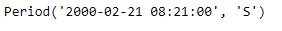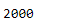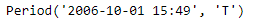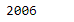Python | Pandas Period.qyear

• Last Updated : 06 Jan, 2019

Python is a great language for doing data analysis, primarily because of the fantastic ecosystem of data-centric python packages. Pandas is one of those packages and makes importing and analyzing data much easier.

Pandas Period.qyear attribute return the fiscal year the Period lies in according to its starting-quarter. The year and the qyear of the period will be the same if the fiscal and calendar years are the same. When they are not, the fiscal year can be different from the calendar year of the period.

Syntax : Period.qyear

Parameters : None

Return : fiscal year

Example #1: Use Period.qyear attribute to find the fiscal year the period lies in for the given Period object.

 # importing pandas as pdimport pandas as pd  # Create the Period objectprd = pd.Period(freq ='S', year = 2000, month = 2,                  day = 21, hour = 8, minute = 21)  # Print the Period objectprint(prd)

Output :Now we will use the Period.qyear attribute to find the fiscal year

 # return the fiscal yearprd.qyear

Output :As we can see in the output, the Period.qyear attribute has returned 2000 indicating that the given period lies in the year of 2000.

Example #2: Use Period.qyear attribute to find the fiscal year the period lies in for the given Period object.

 # importing pandas as pdimport pandas as pd  # Create the Period objectprd = pd.Period(freq ='T', year = 2006, month = 10,                            hour = 15, minute = 49)  # Print the Period objectprint(prd)

Output :Now we will use the Period.qyear attribute to find the fiscal year

 # return the fiscal yearprd.qyear

Output :As we can see in the output, the Period.qyear attribute has returned 2006 indicating that the given period lies in the year of 2006.

My Personal Notes arrow_drop_up